# Analyze Linearized DSGE Models

This example shows how to analyze the dynamic stochastic general equilibrium (DSGE) model in  using Bayesian state-space model (SSM) tools available in Econometrics Toolbox™. The example shows how to perform the following tasks:

1. Simulate data from a known SSM.

2. Estimate posterior moments of the model parameters by combining prior distributions and the simulated data.

3. Characterize the dynamic behavior of the model by using impulse response and forecast error variance analyses.

4. Forecast response variables by using posterior predictive distribution.

DSGE models explain and predict the co-movement of macroeconomic time series by the long-term strategies of households, firms, and policy makers. DSGE models have a solid microeconomic foundation; they are widely used by central banks for economic forecasting and assessing the macroeconomic impact of the monetary and fiscal policies.

Using DSGE to model macroeconomic time series involves two main steps: solution and estimation. The solution step solves intertemporal optimization problems by applying dynamic programming and numeric approximation techniques. A solved DSGE model has a set of dynamic equations in state-space form. The estimation step fits unknown variables (for example, parameters, states, unobserved series, and future variables) in the model to data by applying likelihood-based classical or Bayesian methods. This example introduces of the econometric analysis of a solved and linearized DSGE model, and also focuses on the following statistical inference applications:

### Model Specification

A new, stylized Keynesian DSGE model economy with households, intermediate goods producers, final goods producers, and monetary and fiscal authorities is described in . The model is a simplified version of the Smets and Wouters model . Larger DSGE models with richer dynamics, for example, the Federal Reserve Bank of New York (FRBNY) DSGE , require a practical approach to obtain a model for parameter estimation:

1. Log-linearize the equilibrium conditions.

2. Solve the linear rational expectations models by using the method described in .

3. Obtain a linear SSM.

The stylized model has a closed-form solution. A summary of the log-linearized equilibrium conditions is in , Table 4, but the equations involved follow here.

Let ${\mathit{x}}_{\mathit{t}}$ be a multivariate time series variable that tracks the following latent econometric states:

• Preference shock

• Price mark-up shock

• Technology growth shock

• Monetary policy shock

• Output log-deviations from the steady states

Let ${\mathit{Y}}_{\mathit{t}}$ be a multivariate time series variable for the following observations:

• Output growth

• Labor share

• Inflation rate

• Interest rate

The model is specified by the state and measurement equations:

• State equation: ${\mathit{x}}_{\mathit{t}}={\mathit{A}}_{1}{\mathit{x}}_{\mathit{t}-1}+{\mathit{B}}_{1}{\mathit{u}}_{\mathit{t}}$,

• Measurement equation: ${\mathit{Y}}_{\mathit{t}}={\mathit{C}}_{0}+{\mathit{C}}_{1}{\mathit{x}}_{\mathit{t}}$,

where

• ${\mathit{A}}_{1}=\left[\begin{array}{ccccc}{\rho }_{\varphi }& 0& 0& 0& 0\\ 0& {\rho }_{\lambda }& 0& 0& 0\\ 0& 0& {\rho }_{\mathit{z}}& 0& 0\\ 0& 0& 0& 0& 0\\ {\mathit{a}}_{\varphi \text{\hspace{0.17em}}}& {\mathit{a}}_{\lambda \text{\hspace{0.17em}}}& {\mathit{a}}_{\mathit{z}}& {\mathit{a}}_{\mathit{R}}& 0\end{array}\right]$.

• ${\mathit{B}}_{1}=\left[\begin{array}{cccc}{\sigma }_{\varphi }& 0& 0& 0\\ 0& {\sigma }_{\lambda }& 0& 0\\ 0& 0& {\sigma }_{\mathit{z}}& 0\\ 0& 0& 0& 1\\ 0& 0& 0& 0\end{array}\right]$.

• ${\mathit{C}}_{0}=\left[\begin{array}{c}\mathrm{log}\left(\gamma \text{\hspace{0.17em}}\right)\\ \mathrm{log}\left(\mathit{h}\right)\\ \mathrm{log}\left({\pi }^{*}\text{\hspace{0.17em}}\right)\\ \mathrm{log}\left(\frac{{\pi }^{*}\gamma \text{\hspace{0.17em}}}{\beta \text{\hspace{0.17em}}}\right)\end{array}\right]$.

• ${\mathit{C}}_{1}=\left[\begin{array}{ccccc}{\mathit{a}}_{\varphi }& {\mathit{a}}_{\lambda \text{\hspace{0.17em}}}& {\mathit{a}}_{\mathit{z}}+1& {\mathit{a}}_{\mathit{R}}& -1\\ 1+\left(1+\mathit{v}\right){\mathit{a}}_{\varphi }& \left(1+\mathit{v}\right){\mathit{a}}_{\lambda \text{\hspace{0.17em}}}& \left(1+\mathit{v}\right){\mathit{a}}_{\mathit{z}}& \left(1+\mathit{v}\right){\mathit{a}}_{\mathit{R}}& 0\\ \frac{{\mathit{k}}_{\mathit{p}}}{1-\beta {\rho }_{\varphi }}\left(1+\left(1+\mathit{v}\right){\mathit{a}}_{\varphi }\right)& \frac{{\mathit{k}}_{\mathit{p}}}{1-\beta {\rho }_{\lambda }}\left(1+\left(1+\mathit{v}\right){\mathit{a}}_{\lambda \text{\hspace{0.17em}}}\right)& \frac{{\mathit{k}}_{\mathit{p}}}{1-\beta {\rho }_{\mathit{z}}}\left(1+\mathit{v}\right){\mathit{a}}_{\mathit{z}}& {\mathit{k}}_{\mathit{p}}\left(1+\mathit{v}\right){\mathit{a}}_{\mathit{R}}& 0\\ \frac{{\mathit{k}}_{\mathit{p}}/\beta }{1-\beta {\rho }_{\varphi }}\left(1+\left(1+\mathit{v}\right){\mathit{a}}_{\varphi }\right)& \frac{{\mathit{k}}_{\mathit{p}}/\beta }{1-\beta {\rho }_{\lambda }}\left(1+\left(1+\mathit{v}\right){\mathit{a}}_{\lambda \text{\hspace{0.17em}}}\right)& \frac{{\mathit{k}}_{\mathit{p}}/\beta }{1-\beta {\rho }_{\mathit{z}}}\left(1+\mathit{v}\right){\mathit{a}}_{\mathit{z}}& {\mathit{k}}_{\mathit{p}}\left(1+\mathit{v}\right){\mathit{a}}_{\mathit{R}}/\beta +{\sigma }_{\mathit{R}}& 0\end{array}\right]$.

• ${\mathit{a}}_{\varphi \text{\hspace{0.17em}}}=-\frac{{\mathit{k}}_{\mathit{p}}{\psi }_{\mathit{p}}/\beta \text{\hspace{0.17em}}}{1-{\psi }_{\mathit{p}}{\rho }_{\varphi }}$.

• ${\mathit{a}}_{\lambda \text{\hspace{0.17em}}}=-\frac{{\mathit{k}}_{\mathit{p}}{\psi }_{\mathit{p}}/\beta \text{\hspace{0.17em}}}{1-{\psi }_{\mathit{p}}{\rho }_{\lambda }}$.

• ${\mathit{a}}_{\mathit{z}\text{\hspace{0.17em}}}=\frac{{\rho }_{\mathit{z}}\text{\hspace{0.17em}}{\psi }_{\mathit{p}}}{1-{\psi }_{\mathit{p}}{\rho }_{\varphi }}$.

• ${\mathit{a}}_{\mathit{R}}=-{\psi }_{\mathit{p}}{\sigma }_{\mathit{R}}$.

• $\mathit{h}=\frac{1}{1+\lambda }$.

• ${\mathit{k}}_{\mathit{p}}=\frac{\left(1-{\zeta }_{\mathit{p}}\beta \right)\left(1-{\zeta }_{\mathit{p}}\right)}{{\zeta }_{\mathit{p}}}$.

• ${\psi }_{\mathit{p}}={\left[1+\frac{{\mathit{k}}_{\mathit{p}}}{\beta }\left(1+\mathit{v}\right)\right]}^{-1}$.

The model parameters are $\theta =\left(\beta ,\gamma ,\lambda ,{\pi }^{*},{\zeta }_{\mathit{p}},\mathit{v},{\rho }_{\varphi },{\rho }_{\lambda },{\rho }_{\mathit{z}},{\sigma }_{\varphi },{\sigma }_{\lambda },{\sigma }_{\mathit{z}},{\sigma }_{\mathit{R}}\right)$.

Econometrics Toolbox SSM software defines a state-space model by the coefficient matrices $\mathit{A}$, $\mathit{B}$, $\mathit{C}$, and $\mathit{D}$ such that

${\mathit{X}}_{\mathit{t}}=\mathit{A}\text{\hspace{0.17em}}{\mathit{X}}_{\mathit{t}-1}+\mathit{B}\text{\hspace{0.17em}}{\mathit{u}}_{\mathit{t}}$

${\mathit{Y}}_{\mathit{t}}=\mathit{C}\text{\hspace{0.17em}}{\mathit{X}}_{\mathit{t}}+\mathit{D}\text{\hspace{0.17em}}{\epsilon }_{\mathit{t}}$,

where

• ${\mathit{X}}_{\mathit{t}}=\left[\begin{array}{c}{\mathit{x}}_{\mathit{t}}\\ 1\end{array}\right]$.

• $\mathit{A}=\left[\begin{array}{cc}{\mathit{A}}_{1}& 0\\ 0& 1\end{array}\right]$.

• $\mathit{B}=\left[\begin{array}{c}{\mathit{B}}_{1}\\ 0\end{array}\right]$.

• $\mathit{C}=\left[\begin{array}{cc}{\mathit{C}}_{1}& {\mathit{C}}_{0}\end{array}\right]$.

• $\mathit{D}=0$.

In the state-space form, the constant one in the state vector accommodates an intercept term in the state and measurement equations.

The supporting function Example_dsge2ssm.m converts DSGE equations to an SSM. The input params is a vector of parameters $\theta$ and the outputs are the SSM coefficient matrices $\mathit{A}$, $\mathit{B}$, $\mathit{C}$, and $\mathit{D}$.

Create an SSM object by passing a function handle for Example_dsge2ssm.m to the ssm function.

Mdl = ssm(@Example_dsge2ssm); responses = ["Output growth" "Labor share" "Inflation rate" "Interest rate"];
function [A,B,C,D] = Example_dsge2ssm(params) % Define state-space model coefficients A, B, C, D, given a specific % parameter vector. % Structural parameters in the DSGE model beta = params(1); gamma = params(2); lambda = params(3); pi = params(4); zeta = params(5); v = params(6); rhoPhi = params(7); rhoLambda = params(8); rhoZ = params(9); sigmaPhi = params(10); sigmaLambda = params(11); sigmaZ = params(12); sigmaR = params(13); % DSGE equilibrium conditions in a linear dynamic form h = 1/(1+lambda); kp = (1-zeta*beta)*(1-zeta)/zeta; psi = 1/(1+kp*(1+v)/beta); aPhi = -kp*psi/beta/(1-psi*rhoPhi); aLambda = -kp*psi/beta/(1-psi*rhoLambda); aZ = rhoZ*psi/(1-psi*rhoZ); aR = -psi*sigmaR; C0 = [log(gamma); log(h); log(pi); log(pi*gamma/beta)]; C1 = [aPhi aLambda (aZ+1) aR -1; 1+(1+v)*aPhi (1+v)*aLambda (1+v)*aZ (1+v)*aR 0; kp/(1-beta*rhoPhi)*(1+(1+v)*aPhi) kp/(1-beta*rhoLambda)*(1+(1+v)*aLambda) kp/(1-beta*rhoZ)*(1+v)*aZ kp*(1+v)*aR 0; kp/beta/(1-beta*rhoPhi)*(1+(1+v)*aPhi) kp/beta/(1-beta*rhoLambda)*(1+(1+v)*aLambda) kp/beta/(1-beta*rhoZ)*(1+v)*aZ kp*(1+v)*aR/beta+sigmaR 0]; A1 = diag([rhoPhi rhoLambda rhoZ 0 0]); A1(end,1:4) = [aPhi aLambda aZ aR]; B1 = diag([sigmaPhi sigmaLambda sigmaZ 1 0]); B1 = B1(:,1:4); % Convert DSGE to SSM coefficients A = blkdiag(A1,1); B = [B1; zeros(1,4)]; C = [C1 C0]; D = zeros(4,0); end 

Mdl is an ssm object representing the SSM.

### Likelihood-Based Inference and Kalman Filter

For a parametric model like the Gaussian linear SSM, the likelihood function is crucial for both frequentist and Bayesian inference. The SSM has the unobserved state variables, the observed measurement variables, and the structural parameters in the transition and measurement equations. The likelihood function is the joint density of measurement variables as a function of the parameters with the state variables integrated out. For a Gaussian linear SSM, the Kalman filter evaluates the exact likelihood function.

Simulate artificial data generated by an SSM, in which the true parameters are in , Table 5.

rng("default") numObs = 100; params = [1/1.01; exp(0.005); 0.15; exp(0.005); 0.65; 0; ... 0.94; 0.88; 0.13; 0.01; 0.01; 0.01; 0.01]; [Y,TrueStates] = simulate(Mdl,numObs,Params=params);

Profile the likelihood function with respect to the Calvo parameter ${\zeta }_{\mathit{p}}$ and fix the remaining parameters at their true values.

numPoints = 200; zetaGrid = linspace(0.5,0.75,numPoints); logLGrid = zeros(1,numPoints); theta = params; for n = 1:numPoints theta(5) = zetaGrid(n); [~,logLGrid(n)] = filter(Mdl,Y,Params=theta); end figure plot(zetaGrid,logLGrid); xline(params(5),"--r","Truth"); xlabel("Calvo Parameter"); ylabel("Loglikelihood"); title("Profile Likelihood Function of Calvo Parameter");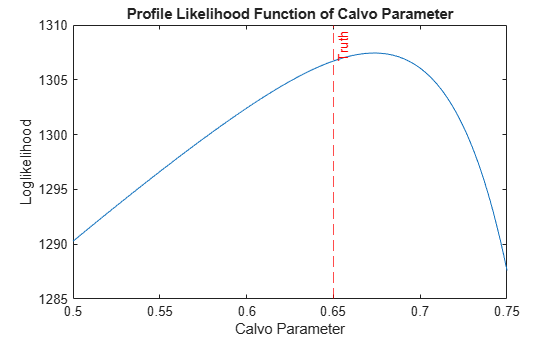The profile likelihood function of the Calvo parameter is hump shaped.

Perform a grid search for the optimal parameter that maximizes the profile likelihood function $\stackrel{ˆ}{\text{\hspace{0.17em}}{\zeta }_{\mathit{p}}}$.

[~,ind] = max(logLGrid); fprintf("Calvo parameter: profile estimator = %4.3f; true value = %4.3f.\n", ... zetaGrid(ind),params(5))
Calvo parameter: profile estimator = 0.673; true value = 0.650. 

The maximizer is reasonably close to the true parameter value. However, the likelihood surface of high dimensional parameters can be complicated, which complicates maximum likelihood estimation (MLE) of the DSGE model. Therefore, the SSM functionality of Econometrics Toolbox uses the Optimization Toolbox functions fminunc or fmincon for numerical MLE.

params0 = [0.99; 1.01; 0.1; 1.01; 0.7; 0; 0.9; 0.7; 0.1; 0.01; 0.01; 0.01; 0.01]; [EstMdl,estParamsMLE] = estimate(Mdl,Y,params0,Univariate=true);
Method: Maximum likelihood (fminunc) Sample size: 100 Logarithmic likelihood: 1314.14 Akaike info criterion: -2602.27 Bayesian info criterion: -2568.41 | Coeff Std Err t Stat Prob --------------------------------------------------------- c(1) | 0.98995 0.00188 525.46723 0 c(2) | 1.00485 0.00126 795.88895 0 c(3) | 0.13863 0.00520 26.68147 0 c(4) | 1.00279 0.00770 130.22893 0 c(5) | 0.68027 0.05136 13.24601 0 c(6) | 0.01469 0.08107 0.18126 0.85617 c(7) | 0.91422 0.03195 28.61191 0 c(8) | 0.71871 0.08503 8.45209 0 c(9) | 0.14771 0.06112 2.41651 0.01567 c(10) | 0.01037 0.00099 10.48757 0 c(11) | 0.01590 0.00543 2.92751 0.00342 c(12) | 0.00952 0.00106 8.98332 0 c(13) | 0.01096 0.00085 12.89207 0 | | Final State Std Dev t Stat Prob x(1) | 0.01695 0 Inf 0 x(2) | -0.02648 0.00000 -3.21629e+08 0 x(3) | -0.00871 0.00000 -4.73181e+07 0 x(4) | -0.40891 0 -Inf 0 x(5) | -0.00738 0.00000 -3.40022e+09 0 x(6) | 1 0 Inf 0 

The main use of the Kalman filter is state estimation; the likelihood evaluation is a by-product. In the forward recursion, the Kalman filter predicts the states based on the current information set, and then it updates the state distribution as it processes new observations. The Kalman filter operates most efficiently when it processes one data point at a time in the presence of vector-valued observations, namely, the univariate treatment of multivariate series (Univariate=true setting).

Estimate the state of the preference shock by the Kalman filter.

States = filter(EstMdl,Y,Univariate=true); figure plot(1:numObs,States(:,1),"-b",1:numObs,TrueStates(:,1),"--r") legend("Estimated","Truth") xlabel("Period"); ylabel("States"); title("State Estimation by the Kalman Filter");The Kalman filter accommodates missing observations (denoted by NaN values). You can estimate the states by using only observations of the output growth, but the results can provide an inaccurate estimate on the state of the preference shock. However, observations of the output growth, the labor share, and the inflation rate jointly provide sharp state estimates.

Specify NaN values for unused observations, and then run the Kalman filter using the partially observed data.

Y1 = Y; Y1(:,2:4) = NaN; States1 = filter(EstMdl,Y1); Y123 = Y; Y123(:,4) = NaN; States123 = filter(EstMdl,Y123); figure tiledlayout(2,1) nexttile plot(1:numObs,States1(:,1),"-b",1:numObs,TrueStates(:,1),"--r"); title("Kalman Filter with One Series") xlabel("Period") ylabel("States") nexttile plot(1:numObs,States123(:,1),"-b",1:numObs,TrueStates(:,1),"--r") title("Kalman Filter with Three Series") xlabel("Period") ylabel("States")### Bayesian Parameter Estimation

Because macroeconomists have expert opinions on values to use in the prior distribution of the model parameters, Bayesian inference is popular in empirical DSGE studies. Bayesian techniques update a prior distribution using sample information in the likelihood function, which leads to the posterior distribution of a parameter. Regularization by the non-sample information in the prior is helpful when the data set lacks adequate information for accurate parameter estimation. For example, the prior can add curvature to a nearly flat likelihood surface or down-weigh regions of the parameter space in conflict with the economic theory.

This example uses a slight variation of the prior distribution in , Table 7. The supporting function Example_dsgePrior.m computes the log prior distribution. The input params is a vector of parameters $\theta$ and the output is the scalar log prior evaluated at $\theta$.

function logPrior = Example_dsgePrior(params) % Posterior log density evaluated at a specific parameter vector. beta = params(1); gamma = params(2); lambda = params(3); pi = params(4); zeta = params(5); v = params(6); rhoPhi = params(7); rhoLambda = params(8); rhoZ = params(9); sigmaPhi = params(10); sigmaLambda = params(11); sigmaZ = params(12); sigmaR = params(13); % 100*(1/beta-1) follows the gamma distribution with mean 0.5 and std % 0.5. densityBeta = 100*beta^(-2)*gampdf(100*(1/beta-1),1,0.5); % 100*log(pi) follows the gamma distribution with mean 1 and std 0.5. densityPi = 100/pi*gampdf(100*log(pi),4,1/4); % 100*log(gamma) follows the normal distribution with mean 0.75 and std % 0.5. densityGamma = 100/gamma*normpdf(100*log(gamma),0.75,0.5); % lambda follows the gamma distribution with mean 0.2 and std 0.2. densityLambda = gampdf(lambda,1,0.2); % zeta follows the beta distribution with mean 0.7 and std 0.15. densityZeta = betapdf(zeta,5.833,2.5); % 1/(1+v) follows the gamma distribution with mean 1.5 and std 0.75. densityV = (1+v)^(-2)*gampdf(1/(1+v),4,0.375); % rho follows the uniform distribution. densityRhoPhi = (rhoPhi >= 0) && (rhoPhi < 1); densityRhoLambda = (rhoLambda >= 0) && (rhoLambda < 1); densityRhoZ = (rhoZ >= 0) && (rhoZ < 1); % sigma follows the inverse gamma distribution. densitySigmaPhi = igpdf(100*sigmaPhi,3/2,1/8)/100; densitySigmaLambda = igpdf(100*sigmaLambda,3/2,2)/100; densitySigmaZ = igpdf(100*sigmaZ,3/2,1/8)/100; densitySigmaR = igpdf(100*sigmaR,3/2,2)/100; % Joint prior distribution logPrior = log(densityBeta) + log(densityPi) + log(densityGamma) ... + log(densityLambda) + log(densityZeta) + log(densityV) ... + log(double(densityRhoPhi)) + log(double(densityRhoLambda)) + log(double(densityRhoZ)) ... + log(densitySigmaPhi) + log(densitySigmaLambda) + log(densitySigmaZ) + log(densitySigmaR); if isnan(logPrior) || ~isreal(logPrior) logPrior = -Inf; end % Inverse gamma density function density = igpdf(x,a,b) logDensity = -gammaln(a)-a.*log(b)-(a+1).*log(x)-1./(b.*x); density = exp(logDensity); end end 

The posterior does not belong to a common distribution family, but the posterior density can be evaluated up to a normalizing constant as the product of the prior and the likelihood.

The random walk Metropolis-Hastings (RWMH) sampler is a popular MCMC method that generates asymptotic samples from the high dimensional posterior. The RWMH sampler first generates a parameter draw from a proposal distribution centered at the current state of the Markov chain. If the posterior density evaluated at the proposed parameter is greater than the current density value, the proposal is accepted. Otherwise, it is accepted with a certain probability to ensure reversibility of the Markov chain. To ensure the RWMH sampler works well, you must carefully tune the proposal. The key tuning parameter of the algorithm is the covariance matrix of the proposal distribution in the form of multivariate normal or $\mathit{t}$ distributions. The inverse of the negative Hessian evaluated at the posterior mode (that is, the maximum of the posterior density) is a natural choice for the covariance matrix, despite the fact that the numeric Hessian is notoriously inaccurate and computationally intensive.

A practical Bayesian parameter estimation workflow is:

1. Create a Bayesian SSM object.

2. Tune the RWMH sampler.

3. Run the tuned RWMH sampler.

#### Create Bayesian SSM Object

Create a Bayesian SSM object by passing function handles to the SSM parameter mapping function Example_dsge2ssm.m and the log prior specification Example_dsgePrior.m to the bssm function.

MdlB = bssm(@Example_dsge2ssm,@Example_dsgePrior);

MdlB is a bssm object representing the Bayesian SSM.

#### Tune RWMH Sampler

Tune the RWMH sampler using numerical optimization of the posterior density by passing the Bayesian SSM object, response data, and initial parameter values to the tune function. Specify the univariate treatment of the multivariate series.

[params0,Proposal] = tune(MdlB,Y,params0,Univariate=true);
Local minimum found. Optimization completed because the size of the gradient is less than the value of the optimality tolerance. Optimization and Tuning | Params0 Optimized ProposalStd ----------------------------------------- c(1) | 0.9900 0.9905 0.0016 c(2) | 1.0100 1.0052 0.0011 c(3) | 0.1000 0.1366 0.0042 c(4) | 1.0100 1.0063 0.0033 c(5) | 0.7000 0.6664 0.0472 c(6) | 0 0.0385 0.0857 c(7) | 0.9000 0.9125 0.0260 c(8) | 0.7000 0.7516 0.0813 c(9) | 0.1000 0.1227 0.0583 c(10) | 0.0100 0.0108 0.0010 c(11) | 0.0100 0.0137 0.0046 c(12) | 0.0100 0.0101 0.0010 c(13) | 0.0100 0.0109 0.0008 

params0 is the posterior mode and Proposal is an approximated inverse Hessian to use as the proposal covariance matrix for the RWMH sampler.

#### Run Tuned RWMH Sampler

Update the posterior distribution by running the RWMH sampler with the Hessian-based proposal (reduced by 75%). Specify a total of 5000 retained draws, a burn-in period of 1000, and the univariate treatment of a multivariate series

[Params,accept] = simulate(MdlB,Y,params0,Proposal/4, ... NumDraws=5000,BurnIn=1000,Univariate=true); estParams = mean(Params,2); EstParamsCov = cov(Params'); fprintf("RWMH sampler proposal acceptance rate = %4.3f.\n",accept);
RWMH sampler proposal acceptance rate = 0.335. 

As a rule of thumb, an ideal acceptance rate of the proposal is approximately 25% to 50%.

Run the RWMH sampler again with the sample-covariance-based proposal, and then compare the MCMC statistics.

ParamsNew = simulate(MdlB,Y,params0,EstParamsCov/4, ... NumDraws=5000,BurnIn=1000,Univariate=true); estParamsNew = mean(ParamsNew,2); disp(array2table([estParamsMLE,estParams,estParamsNew], ... VariableNames=["MLE" "MCMC1" "MCMC2"],RowNames="c(" + (1:13) + ")"))
 MLE MCMC1 MCMC2 _________ ________ ________ c(1) 0.98995 0.99065 0.99049 c(2) 1.0049 1.0054 1.0052 c(3) 0.13863 0.13542 0.13608 c(4) 1.0028 1.0082 1.007 c(5) 0.68027 0.67017 0.68001 c(6) 0.014694 0.039983 0.025718 c(7) 0.91422 0.91587 0.91685 c(8) 0.71871 0.7536 0.75425 c(9) 0.14771 0.12357 0.12161 c(10) 0.010367 0.011134 0.011086 c(11) 0.015903 0.014645 0.015406 c(12) 0.0095232 0.010276 0.010368 c(13) 0.010964 0.01102 0.011035 

Plot the prior and posterior densities of the Calvo parameter ${\zeta }_{\mathit{p}}$.

zetaGrid = linspace(0.3,1,numPoints); priorDensity = betapdf(zetaGrid,5.833,2.5); posteriorDensity = ksdensity(Params(5,:),zetaGrid); figure plot(zetaGrid,posteriorDensity,"-b",zetaGrid,priorDensity,"--r"); legend("Posterior","Prior") title("Prior and Posterior Distributions of Calvo Parameter") xlabel("\zeta_p") ylabel("Density")### Model-Implied Macroeconomic Dynamics

The model-implied correlations between variables, which are the impulse response functions (IRFs) and forecast error variance decomposition (FEVD), can explain the dynamic interactions between macroeconomic variables.

#### Temporal Correlations

Autocorrelations and cross-correlations characterize dynamic relationships between variables over time. In a model with stationary variables, the model-implied temporal moments take the form $\mathrm{corr}\left({\mathit{y}}_{\mathit{t}},{\mathit{y}}_{\mathit{t}-\mathit{h}}\right)$, $\mathrm{corr}\left({\mathit{x}}_{\mathit{t}},{\mathit{x}}_{\mathit{t}-\mathit{h}}\right)$, and $\mathrm{corr}\left({\mathit{y}}_{\mathit{t}},{\mathit{x}}_{\mathit{t}-\mathit{h}}\right)$, where the index $\mathit{h}\ge 0$ is the number of lags. In a stationary system, leads and lags satisfy the identity $\mathrm{corr}\left({\mathit{y}}_{\mathit{i},\mathit{t}},{\mathit{y}}_{\mathit{j},\mathit{t}+\mathit{h}}\right)=\mathrm{corr}\left({\mathit{y}}_{\mathit{j},\mathit{t}},{\mathit{y}}_{\mathit{i},\mathit{t}-\mathit{h}}\right),\forall \text{\hspace{0.17em}}\mathit{i},\mathit{j}$.

Compute the temporal correlations in the fitted SSM. Specify 12 lags.

numLags = 12; CYY = corr(Mdl,Params=estParams,NumLags=numLags);

The output CYY is a three-dimensional array of temporal correlations $\mathrm{corr}\left({\mathit{y}}_{\mathit{i},\mathit{t}},{\mathit{y}}_{\mathit{j},\mathit{t}-\mathit{h}}\right),\forall \mathit{h},\mathit{i},\mathit{j}$.

Plot the autocorrelations of the output growth (top panel) and the cross-correlations between the output growth and the labor share, the inflation, and interest rate (bottom panel).

CYYlag12 = CYY(1:7,1,2); CYYlag13 = CYY(1:7,1,3); CYYlag14 = CYY(1:7,1,4); CYYlead12 = CYY(7:-1:2,2,1); CYYlead13 = CYY(7:-1:2,3,1); CYYlead14 = CYY(7:-1:2,4,1); seq1 = 0:numLags; seq2 = -(numLags/2):(numLags/2); figure tiledlayout(2,1) nexttile plot(seq1,CYY(:,1,1)) legend(responses(1)) title("Model-Implied Correlations") xlabel("Lag") ylabel("Correlation") nexttile plot(seq2,[CYYlead12; CYYlag12],"-b", ... seq2,[CYYlead13;CYYlag13],":r", ... seq2,[CYYlead14;CYYlag14],"--c"); legend(responses(2:end)) title("Model-Implied Correlations") xlabel("Lag") ylabel("Correlation") axis tight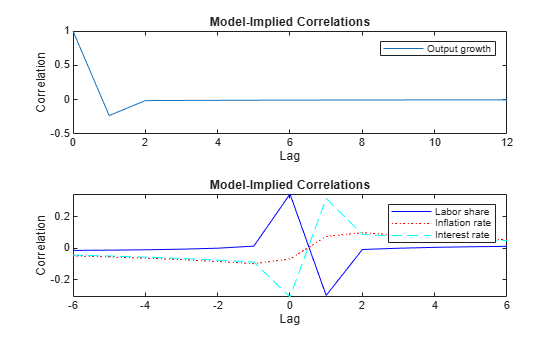Because the response variables ${\mathit{Y}}_{\mathit{t}}$ are observable and the sample analogues are available, the temporal correlation $\mathrm{corr}\left({\mathit{y}}_{\mathit{t}},{\mathit{y}}_{\mathit{t}-\mathit{h}}\right)$ is a key quantity to understanding the dynamics. In a correctly specified model with reasonable parameter estimates, the model-implied moments are close to the empirical moments obtained from the data. You can obtain the empirical moments from the sample analogues or a fitted parametric model, such as a vector autoregression (VAR) model.

Compute and plot the sample correlations of the response variables for 12 lags.

SampleCorr = [crosscorr(Y(:,1),Y(:,1),NumLags=numLags) ... crosscorr(Y(:,2),Y(:,1),NumLags=numLags) ... crosscorr(Y(:,3),Y(:,1),NumLags=numLags) ... crosscorr(Y(:,4),Y(:,1),NumLags=numLags)]'; seq3 = -numLags:numLags; figure tiledlayout(2,1) nexttile plot(seq3,SampleCorr(1,:)) legend(responses(1)) title("Empirical Correlations") xlabel("Lag") ylabel("Correlation") axis tight nexttile plot(seq3,SampleCorr(2,:),"-b",seq3,SampleCorr(3,:),":r", ... seq3,SampleCorr(4,:),"--c") legend(responses(2:end)) title("Empirical Correlations") xlabel("Lag") ylabel("Correlation") axis tight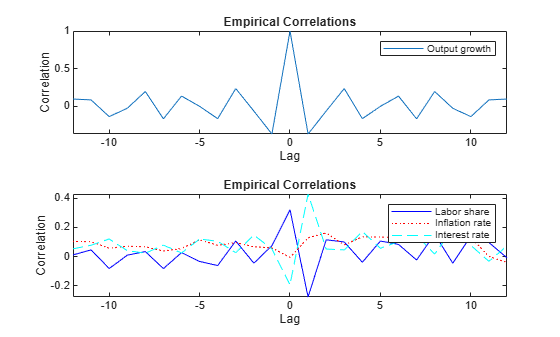#### Impulse Response Functions

Another approach to model-dynamics characterization is impulse response analysis, which studies how the dynamic system responds to a unit impulse of the structural shock, all other conditions being equal. You can obtain the IRF from the moving-average representation of an SSM

${\mathit{y}}_{\mathit{t}}={\Psi }_{0}+{\Psi }_{1}\sum _{\mathit{s}=0}^{\infty }{\Phi }_{1}^{\mathit{s}}{\Phi }_{ϵ}{ϵ}_{\mathit{t}-\mathit{s}}$.

The coefficient ${\Psi }_{1}{\Phi }_{1}^{\mathit{s}}{\Phi }_{ϵ}$ demonstrates the $\mathit{s}$-step-ahead response after an impulse of a state shock. An alternative to the period-by-period IRF is the sum of the IRF over time, which gives the cumulative effect. In the stylized new Keynesian DSGE model, the first element of ${\mathit{Y}}_{\mathit{t}}$ is the single-period output growth. The interpretation of its cumulative IRF is the multiple-period output growth due to additivity of the log-returns.

Compute the IRFs by passing the fitted SSM to the irf function. Specify the estimated parameters and their estimated covariance matrix, and a 21-period cumulative IRF.

[Response,~,Lower,Upper] = irf(Mdl,Params=estParams, ... EstParamCov=EstParamsCov,NumPeriods=21,Cumulative=true);

Response is a three-dimensional array in which the element $\left(\mathit{s},\mathit{i},\mathit{j}\right)$ characterizes the $\left(\mathit{s}-1\right)$ period-ahead response of variable $\mathit{j}$ to a unit of shock to variable $\mathit{i}$.

Plot the model-implied cumulative IRF of the output growth (in percentage) in response to the preference shock, the price mark-up shock, the technology growth shock, and the monetary policy shock.

Response = 100*Response; Lower = 100*Lower; Upper = 100*Upper; titles = ["Preference" "Mark-Up" "Technology" "Monetary"] ... + " Shock"; seq4 = 0:20; ylims = [-0.8 0; -0.8 0.1; 0.5 1.5; -0.8, 0.2]; figure tiledlayout(2,2) for j = 1:4 nexttile plot(seq4,Response(:,j,1),"-b",seq4,Lower(:,j,1),"--r", ... seq4,Upper(:,j,1),"--r"); title(titles(j)) xlabel("Period") ylabel("Response") ylim(ylims(j,:)) end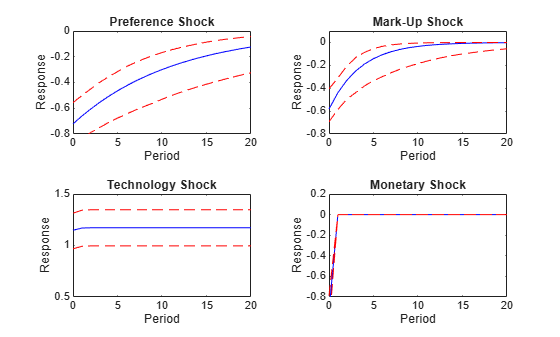#### Forecast Error Variance Decomposition

In addition to temporal correlations and IRFs, variance decompositions are informative about the model dynamics. The FEVD attributes the volatility of observations to component-wise state shocks. It shows the relative importance of each shock in affecting the forecast error variance. You can obtain the FEVD from the moving-average representation of an SSM

${\mathit{y}}_{\mathit{t}}={\Psi }_{0}+{\Psi }_{1}\sum _{\mathit{s}=0}^{\infty }{\Phi }_{1}^{\mathit{s}}{\Phi }_{ϵ}{ϵ}_{\mathit{t}-\mathit{s}}$.

The total variances over $\mathit{h}$ periods are ${\Psi }_{1}\left(\sum _{\mathit{s}=0}^{\mathit{h}-1}{\Phi }_{1}^{\mathit{s}}{\Phi }_{ϵ}{\Phi }_{ϵ}^{\prime }{\Phi }_{1}^{\prime \mathit{s}}\right){\Psi }_{1}^{\prime }$, to which the ${\mathit{j}}^{\mathrm{th}}$ shock contributes ${\Psi }_{1}\left(\sum _{\mathit{s}=0}^{\mathit{h}-1}{\Phi }_{1}^{\mathit{s}}{\Phi }_{\mathit{j},ϵ}{\Phi }_{\mathit{j},ϵ}^{\prime }{\Phi }_{1}^{\prime \mathit{s}}\right){\Psi }_{1}^{\prime }$, where ${\Phi }_{\mathit{j},ϵ}$ is the ${\mathit{j}}^{\mathrm{th}}$ column of the matrix.

Compute the variance decompositions by passing the fitted SSM to the fevd function. Plot the FEVDs in bar graphs.

Decomposition = fevd(Mdl,Params=estParams); figure tiledlayout(2,2) for j = 1:4 nexttile bar(Decomposition(:,:,j),"stacked") title(titles(j)) xlabel("Period") ylabel("Portion") end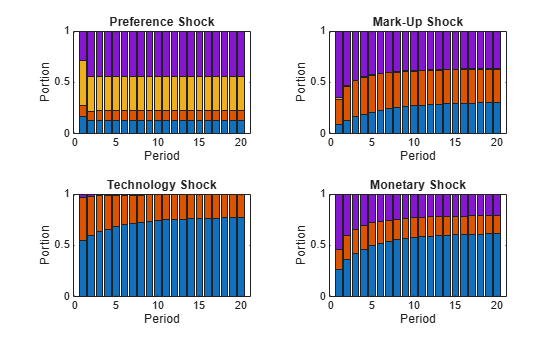The stacked series represent the structural shocks in the state equation, specifically from bottom to top bar, the preference shock, the price mark-up shock, the technology growth shock, and the monetary policy shock. Because the measurement equation is free from additive disturbances, the portions of the four shocks sum to one.

### Simulation-Based Forecast

Bayesian forecasts derive from the posterior predictive distribution $\mathit{p}\left({\mathit{Y}}_{\mathit{f}}|\mathit{Y}\right)=\int \mathit{p}\left({\mathit{Y}}_{\mathit{f}}|\mathit{Y},\theta \right)\mathit{p}\left(\theta |\mathit{Y}\right)\mathit{d}\theta \text{\hspace{0.17em}}$. In the equation, the model parameters are integrated out. Therefore, a Bayesian forecast accounts for parameter uncertainty and the volatility of future data. With the posterior samples obtained by MCMC, the simulation-based forecast computes a collection of predicted variables conditional on the model parameters, and then takes the average of the prediction results. In particular:

• The posterior predictive mean is $\mathit{E}\left({\mathit{Y}}_{\mathit{f}}|\mathit{Y}\right)=\mathit{E}\left[\mathit{E}\left({\mathit{Y}}_{\mathit{f}}|\mathit{Y},\theta \right)|\mathit{Y}\right]$.

• The posterior predictive variance is $\mathrm{Var}\left({\mathit{Y}}_{\mathit{f}}|\mathit{Y}\right)=\mathit{E}\left[\mathrm{Var}\left({\mathit{Y}}_{\mathit{f}}|\mathit{Y},\theta \right)|\mathit{Y}\right]+\mathrm{Var}\left[\mathit{E}\left({\mathit{Y}}_{\mathit{f}}|\mathit{Y},\theta \right)|\mathit{Y}\right]$.

To reduce the computational burden of Monte-Carlo integration, thin the posterior samples by selecting every other 50th draw (column) of Params.

thin = 50; ParamsThin = Params(:,1:thin:end);

Forecast 12 periods out-of-sample using the state-space framework, conditional on the model parameters.

numPeriods = 12; numDraws = size(ParamsThin,2); % Preallocate YfSim = zeros(numPeriods,4,numDraws); YfVarSim = zeros(numPeriods,4,numDraws); for n = 1:numDraws [A,B,C,D] = Example_dsge2ssm(ParamsThin(:,n)); MdlF = ssm(A,B,C,D); [YfSim(:,:,n),YfVarSim(:,:,n)] = forecast(MdlF,numPeriods,Y); end

YfSim is $\mathit{E}\left({\mathit{Y}}_{\mathit{f}}|\mathit{Y},\theta \right)$ and YfVarSim is $\mathrm{Var}\left({\mathit{Y}}_{\mathit{f}}|\mathit{Y},\theta \right)$.

Marginalize over the parameters for the posterior predictive mean and variance.

Yf = mean(YfSim,3); YfVar = mean(YfVarSim,3) + var(YfSim,0,3);

Plot the forecasted values. Use the slider to choose a different response series to plot.

YfLB = Yf - 2*sqrt(YfVar); YfUB = Yf + 2*sqrt(YfVar); ind =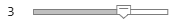3; fh = (numObs+1):(numObs+numPeriods); figure plot(1:numObs,Y(:,ind),":b",fh,Yf(:,ind),"-b", ... fh,YfLB(:,ind),"--r",fh,YfUB(:,ind),"--r") title("Out-of-Sample Forecast of " + responses(ind)) xlabel("Period") ylabel("Value")### Conclusion

Quantitative evaluation of the DSGE model has evolved considerably over the years. Formal parameter estimation is typically implemented in the state-space framework, in which the Kalman filter delivers the likelihood function for the Gaussian linear SSM. It is possible to estimate DSGE models by the numeric maximum likelihood, but Bayesian methods are popular in that a tight prior distribution can regularize the likelihood function. Bayesian inference of the DSGE model is facilitated by MCMC methods such as the Metropolis-Hasting sampler. Appropriate specification of the proposal distribution is crucial for an efficient MCMC. A popular specification is the random walk Gaussian proposal with the covariance matrix proportional to the inverse Hessian evaluated at the posterior maximum. In addition to parameter estimation, it is of interest to estimate other unknown variables such as the state variables, missing data, and the variables in the forecast horizon. In the Bayesian framework, the posterior predictive distribution characterizes the state of knowledge of those unknown variables, conditional on the observations.

The Econometrics Toolbox provides ssm and bssm functionalities for general-purpose state-space modeling. You can cast many time series models, including the linearized DSGE model, in the state-space form for a variety of inference tasks such as maximum likelihood estimation, Bayesian posterior simulation, state filtering and smoothing, impulse response analysis, variance decomposition, out-of-sample forecast, and so on.

 Del Negro, Marco, Eusepi, Stefano, Giannoni, Marc P., Sbordone, Argia M., Tambalotti, Andrea, Cocci, Matthew, Hasegawa, Raiden, and Linder, M. H. "The FRBNY DSGE Model." FRB of New York Staff Report 647 (October 2013). https://doi.org/10.2139/ssrn.2344312.

 Fernández-Villaverde, Jesús, Rubio-Ramírez, Juan F., and Schorfheide, Frank. "Solution and Estimation Methods for DSGE Models." Handbook of Macroeconomics 2 (November 2016) 527–724. https://doi.org/10.1016/bs.hesmac.2016.03.006.

 Sims, Christopher A. "Solving Linear Rational Expectations Models." Computational Economics 20 (October 2002) 1–20. https://doi.org/10.1023/A:1020517101123.

 Smets, Frank, and Raf Wouters. "An Estimated Dynamic Stochastic General Equilibrium Model of the Euro Area." Journal of the European Economic Association 1 (September 2003): 1123–1175. https://doi.org/10.1162/154247603770383415.